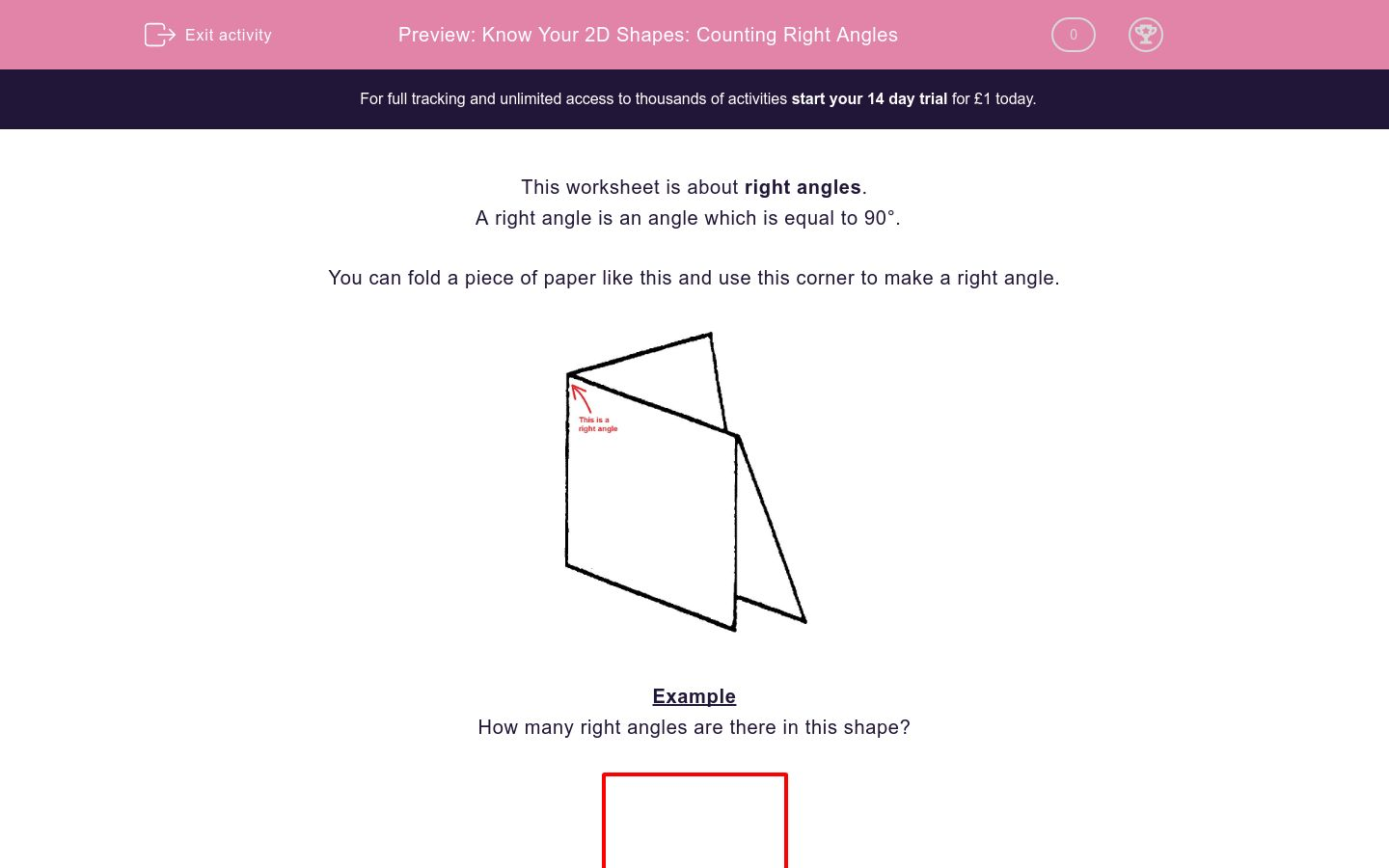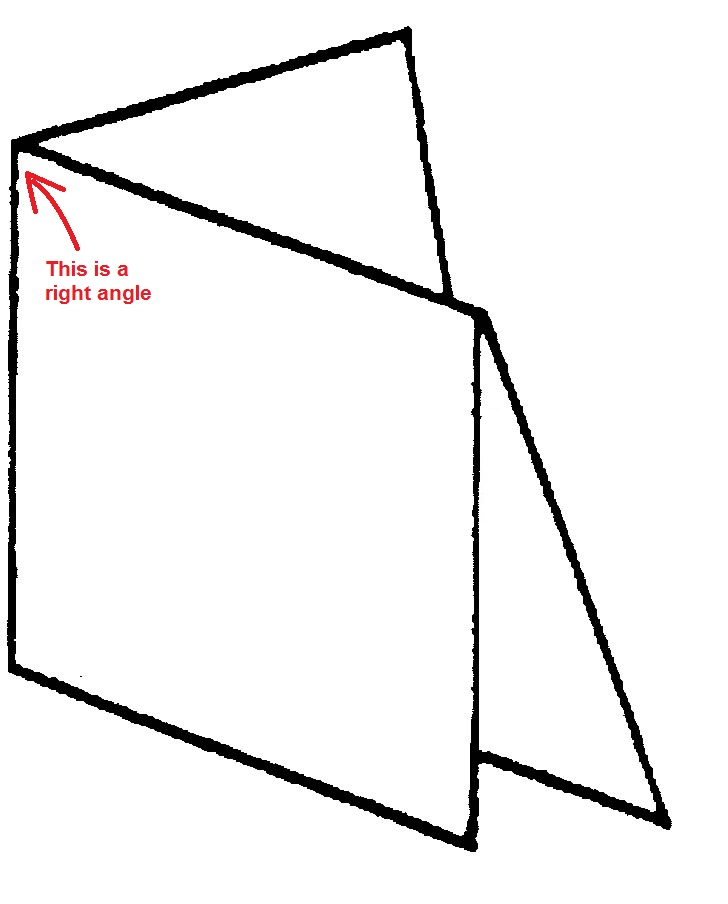# Know Your 2D Shapes: Counting Right Angles

In this worksheet,students count the number of right angles in the 2D shapes shown.Key stage:  KS 2

Curriculum topic:   Maths and Numerical Reasoning

Curriculum subtopic:   2D Shapes: Circles, Angles and Bearings

Difficulty level:### QUESTION 1 of 10

This worksheet is about right angles.

A right angle is an angle which is equal to 90°.

You can fold a piece of paper like this and use this corner to make a right angle.Example

How many right angles are there in this shape?There are 4 right angles, shown in blue.

How many right angles are there in this shape?5

0

6

How many right angles are there in this shape?0

2

3

How many right angles are there in this shape?1

2

3

How many right angles are there in this shape?4

2

3

How many right angles are there in this shape?4

2

3

How many right angles are there in this shape?4

2

3

How many right angles are there in this shape?5

2

0

How many right angles are there in this shape?5

2

0

How many right angles are there in this shape?3

2

5

How many right angles are there inside this shape?6

2

0

• Question 1

How many right angles are there in this shape?0
EDDIE SAYS
None of the angles are right angles.
• Question 2

How many right angles are there in this shape?0
EDDIE SAYS
None of the angles are right angles.
• Question 3

How many right angles are there in this shape?1
EDDIE SAYS• Question 4

How many right angles are there in this shape?4
EDDIE SAYS• Question 5

How many right angles are there in this shape?2
EDDIE SAYS• Question 6

How many right angles are there in this shape?4
EDDIE SAYS• Question 7

How many right angles are there in this shape?0
EDDIE SAYS
There are no right angles in this pentagon.
• Question 8

How many right angles are there in this shape?0
EDDIE SAYS
There are no right angles in this curved shape. It is called an oval or an ellipse.
• Question 9

How many right angles are there in this shape?3
EDDIE SAYS• Question 10

How many right angles are there inside this shape?2
EDDIE SAYS---- OR ----

Sign up for a £1 trial so you can track and measure your child's progress on this activity.

### What is EdPlace?

We're your National Curriculum aligned online education content provider helping each child succeed in English, maths and science from year 1 to GCSE. With an EdPlace account you’ll be able to track and measure progress, helping each child achieve their best. We build confidence and attainment by personalising each child’s learning at a level that suits them.

Get started